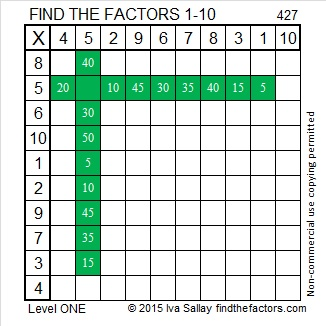# 427 and Level 1

Because one of 427’s factors is the hypotenuse of a primitive Pythagorean triple, 427 is also the hypotenuse of the Pythagorean triple, 77-420-427.

What was that primitive Pythagorean triple? Scroll down past the puzzle to see if you were right.Print the puzzles or type the factors on this excel file:10 Factors 2015-03-16

[77-420-427] is [11-60-61] times seven.

• 427 is a composite number.
• Prime factorization: 427 = 7 x 61
• The exponents in the prime factorization are 1 and 1. Adding one to each and multiplying we get (1 + 1)(1 + 1) = 2 x 2 = 4. Therefore 427 has exactly 4 factors.
• Factors of 427: 1, 7, 61, 427
• Factor pairs: 427 = 1 x 427 or 7 x 61
• 427 has no square factors that allow its square root to be simplified. √427 ≈ 20.6640This site uses Akismet to reduce spam. Learn how your comment data is processed.